• 利用MATLAB设计线性二次型最优控制器-利用MATLAB设计线性二次型最优控制器.pdf 利用MATLAB设计线性二次型最优控制器，一篇论文讲的挺好
• Cqjz.m function [num,den]=cqjz(G,kc,yPm G=tf(G; [mag,pha,w]=bode(G*kc; Mag=20*log10(mag; [Gm,Pm,Wcg,Wcp]=margin(G*kc; %phi=(yPm-getfield(Pm'Wcg*pi/180; phi=(yPm+5-Pm*pi/180; alpha=(1+sin(phi/(1-sin(ph
• 实用文案 下面将根据模糊控制器设计步骤 一步步利用 Matlab 工具箱设计模糊控制器 Matlab 模糊控制工具箱为模糊控制器的设计提供了一种非常便捷的途径 通过它我们不需要进行复杂的模糊化模糊推理及反模糊化运算只...
• matlab开发-TimeDelaySystems的控制器设计。时滞系统的控制器设计Simulink基础
• matlab开发-逆变器端部模糊控制器设计。模糊控制IP
• 介绍了一个关于基于MATLAB模糊控制器设计和仿真的应用实例
• 摘 要 以球杆系统作为主体系统中的小球作为被控对象设计一个模糊控制器 控制小球在...立球杆系统模糊控制规则再对球杆系统进行模糊控制器设计并在?MATLAB?仿 真环境下建立控制器的仿真模型对球杆系统进行仿真测试模糊
• 基于WinCC和Matlab的预测控制器设计pdf,基于WinCC和Matlab的预测控制器设计
• CMAC控制器设计与仿真：在小脑神经网络（CMAC）与PID 并行控制的基础上，提出了一种新型的CMAC 控制器，即 FCMAC 控制器
• 基于MATLAB的PID控制器设计.rar 基于MATLAB的PID控制器设计.rar 基于MATLAB的PID控制器设计.rar基于MATLAB的PID控制器设计.rar
• 基于 MATLAB的 PID 控制器设计 基于 MATLAB的 PID 控制器设计 一 PID 控制简介 PID 控制是最早发展起来的经典控制策略 , 是用于过程控制最有效的 策略之一由于其原理简单技术成在实际应用中较易于整定 , 在工业 控制...
• PID控制器与状态反馈控制器MATLAB教学实例设计(投稿版）.pdf
• 一、设计模糊控制器 1.1 创建项目文件夹 1.2 打开MATLAB  1.3 设计模糊控制器 二、设置控制系统 三、simulink仿真 一、设计模糊控制器 1.1 创建项目文件夹 在此路径如图 1.2 打开MATLAB 打开MATLAB ...
本人菜鸟，初学模糊控制器，为了方便以后查阅写此博文，欢迎初学者借鉴，高手指点！

环境：MATLAB R2012a

目录

一、设计模糊控制器

1.1 创建项目文件夹

1.2 打开MATLAB

1.3 设计模糊控制器

二、设置控制系统

一、设计模糊控制器

1.1 创建项目文件夹

在此路径如图1.2 打开MATLAB

打开MATLAB R2012a切换当前目录为上一步路径，如图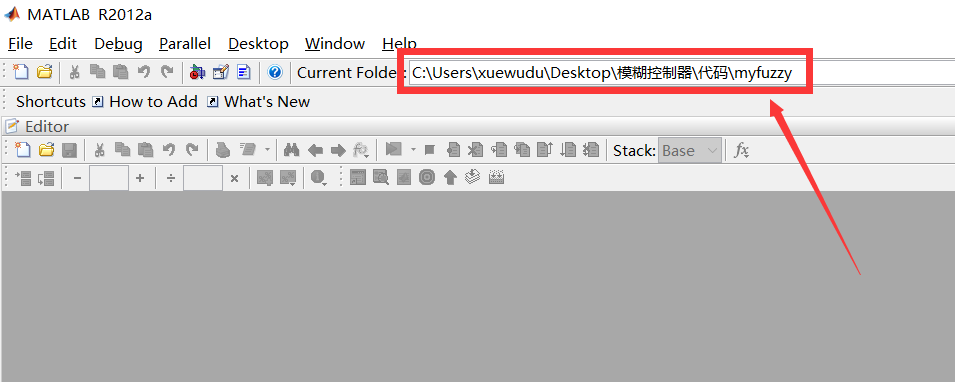1.3 设计模糊控制器

打开模糊控制器设计对话框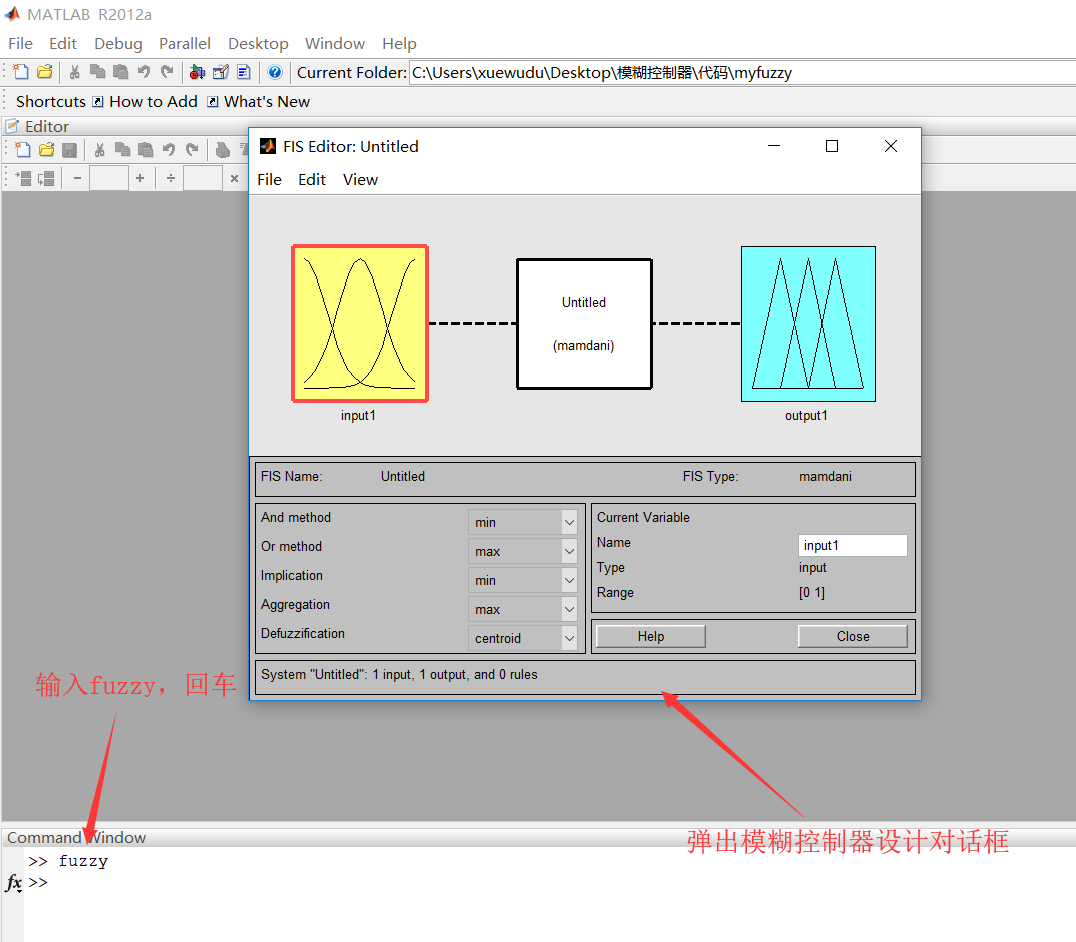根据模糊控制器的输入输出设计模糊控制器，在此以二输入一输出为例。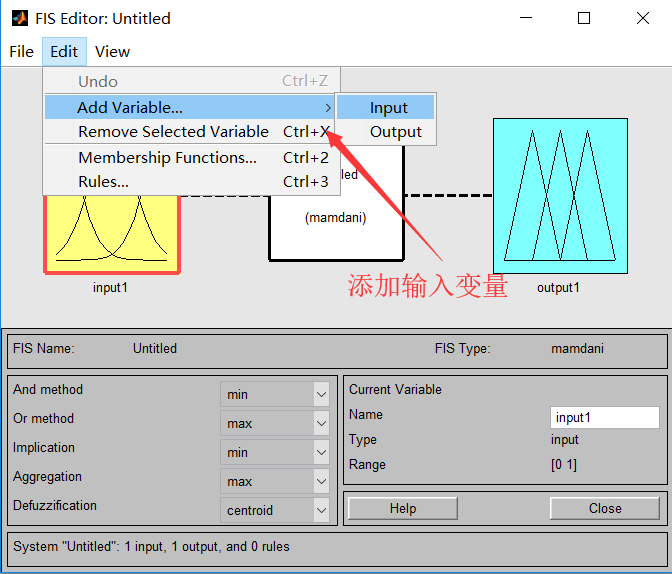完成后如图（左）所示，然后对每个输入输出变量设置隶属函数，如图（右）。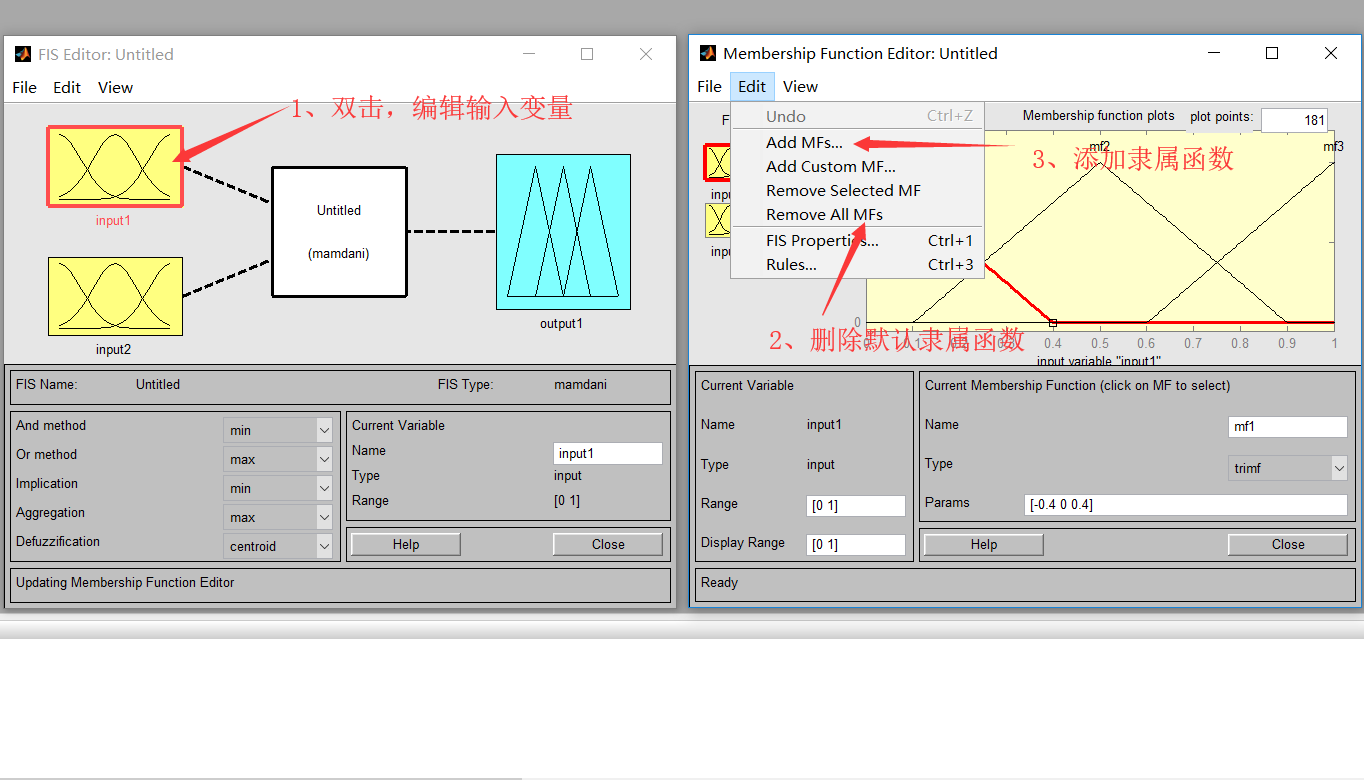添加论域数量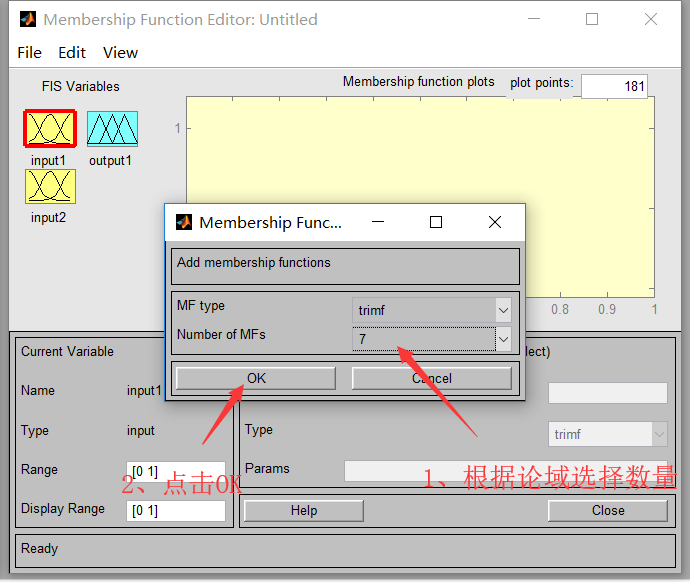设置隶属函数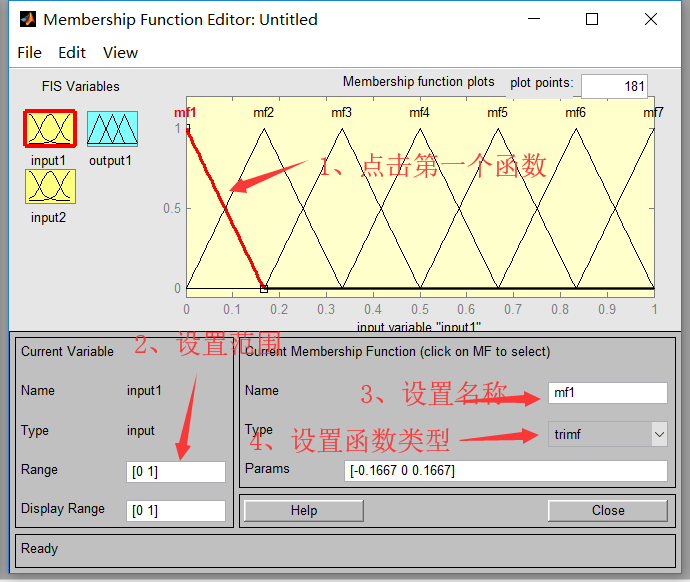完成后如图所示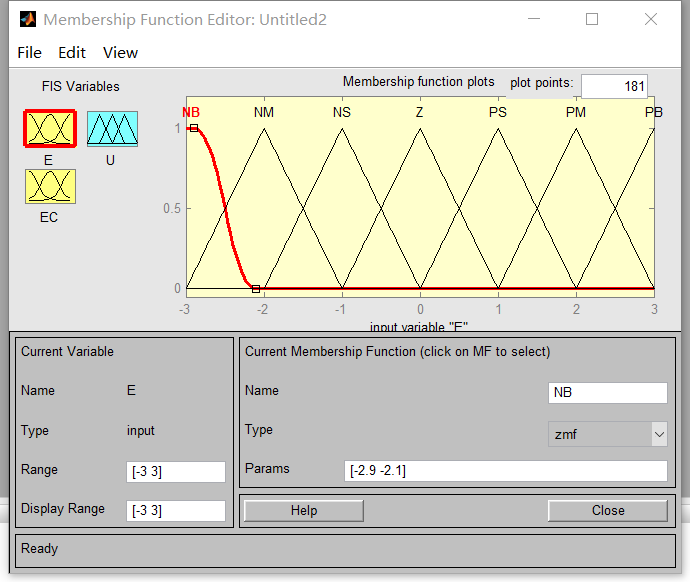设计模糊规则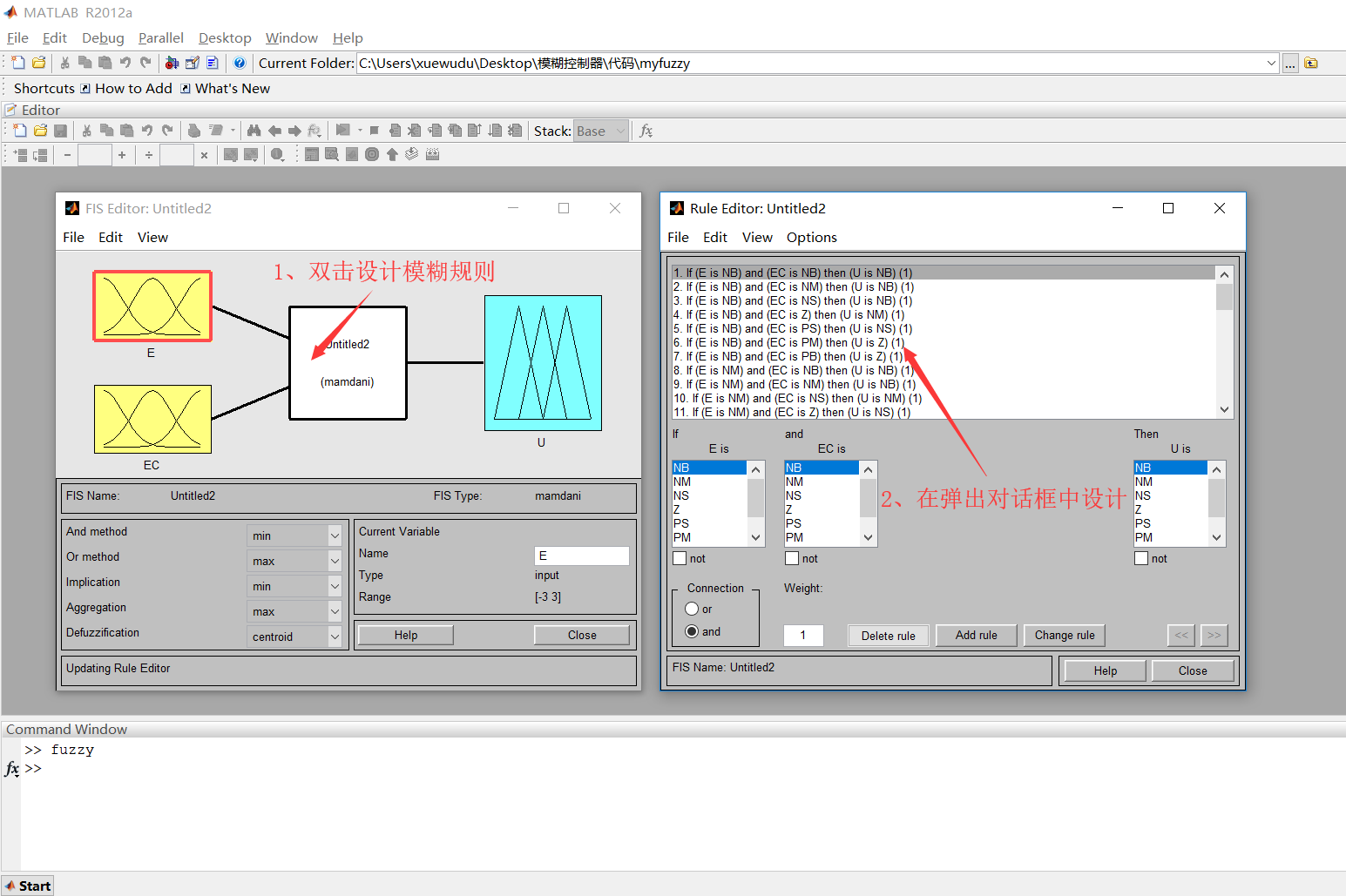保存刚刚设计的模糊控制器，如下图所示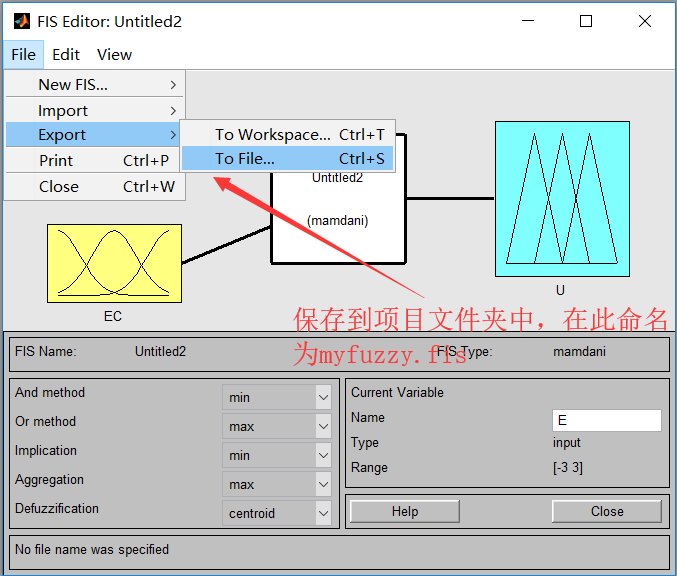加载模糊控制器到MATLAB中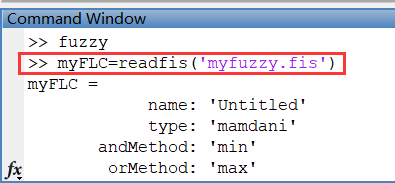二、设置控制系统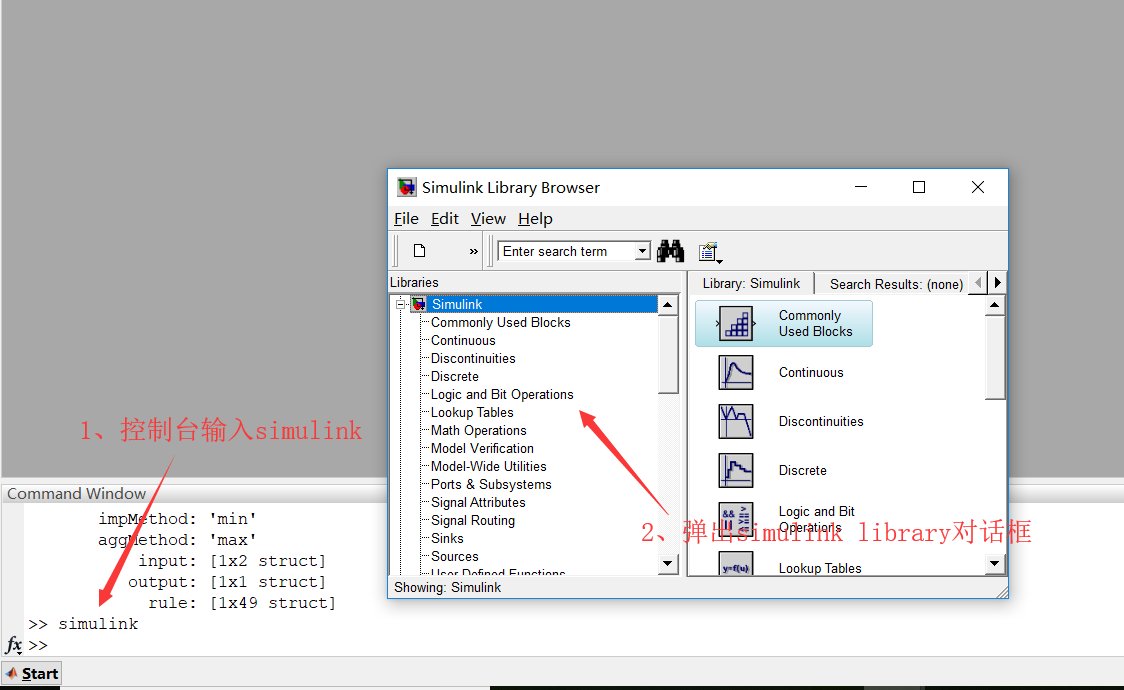设计控制系统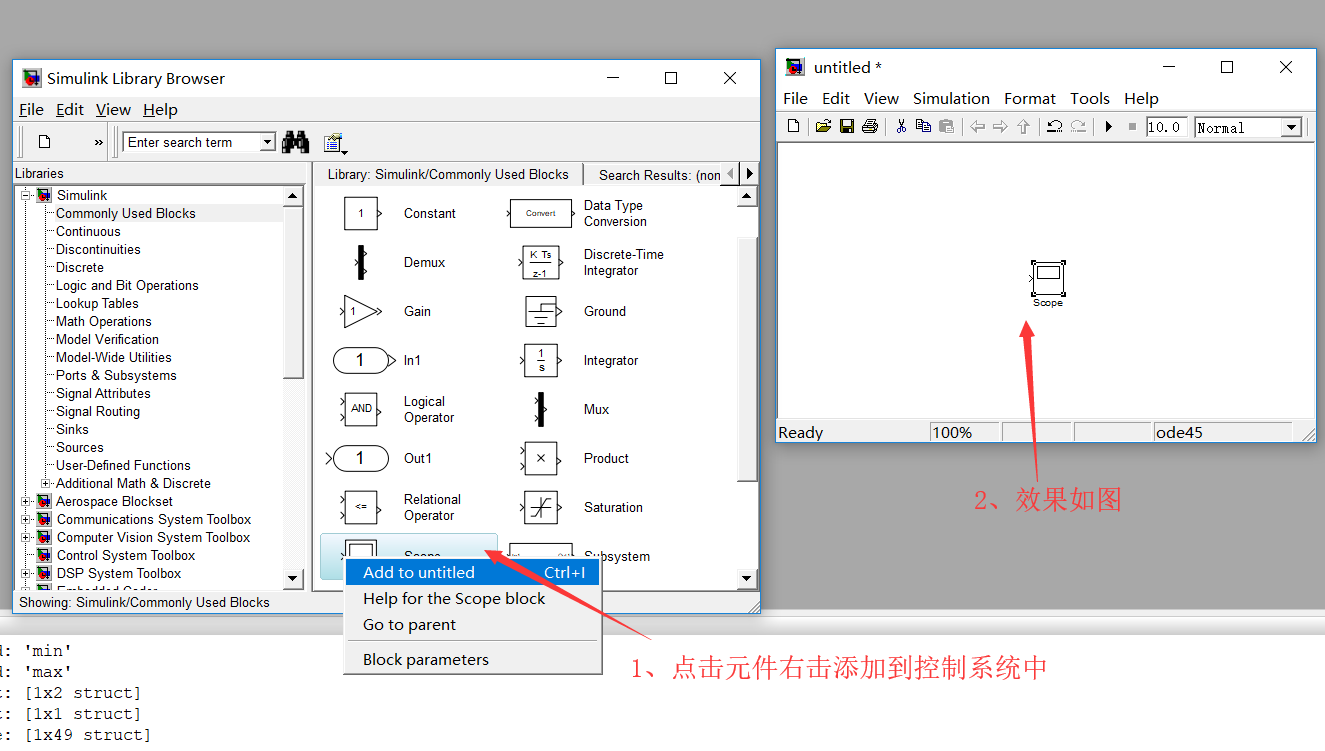设计完成如图所示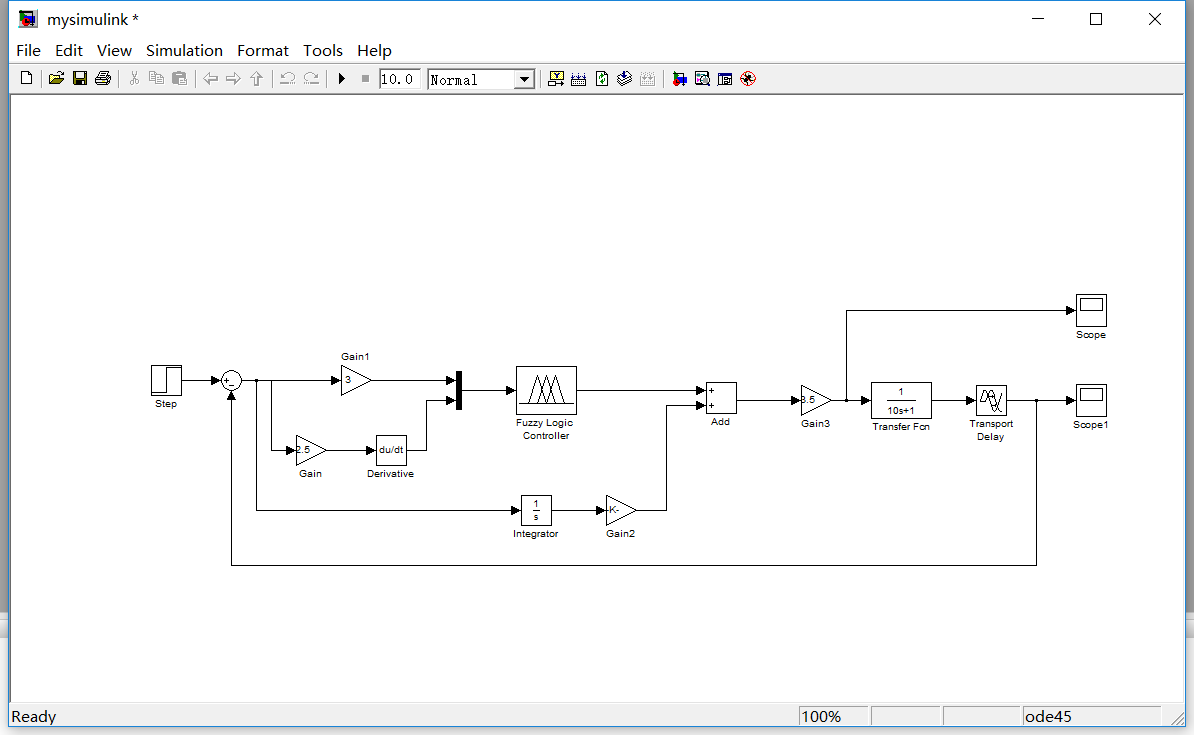添加第一节中设计的模糊控制器，如下图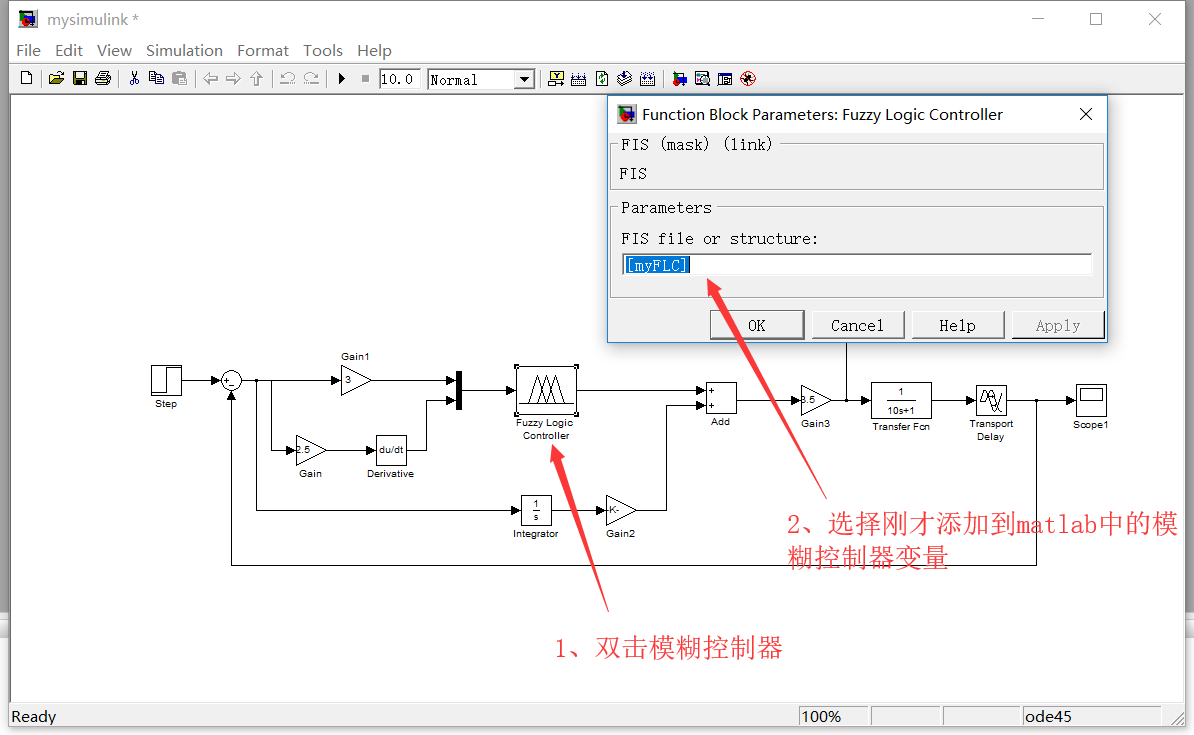自此控制系统设计结束

在仿真之前需要进行如下设置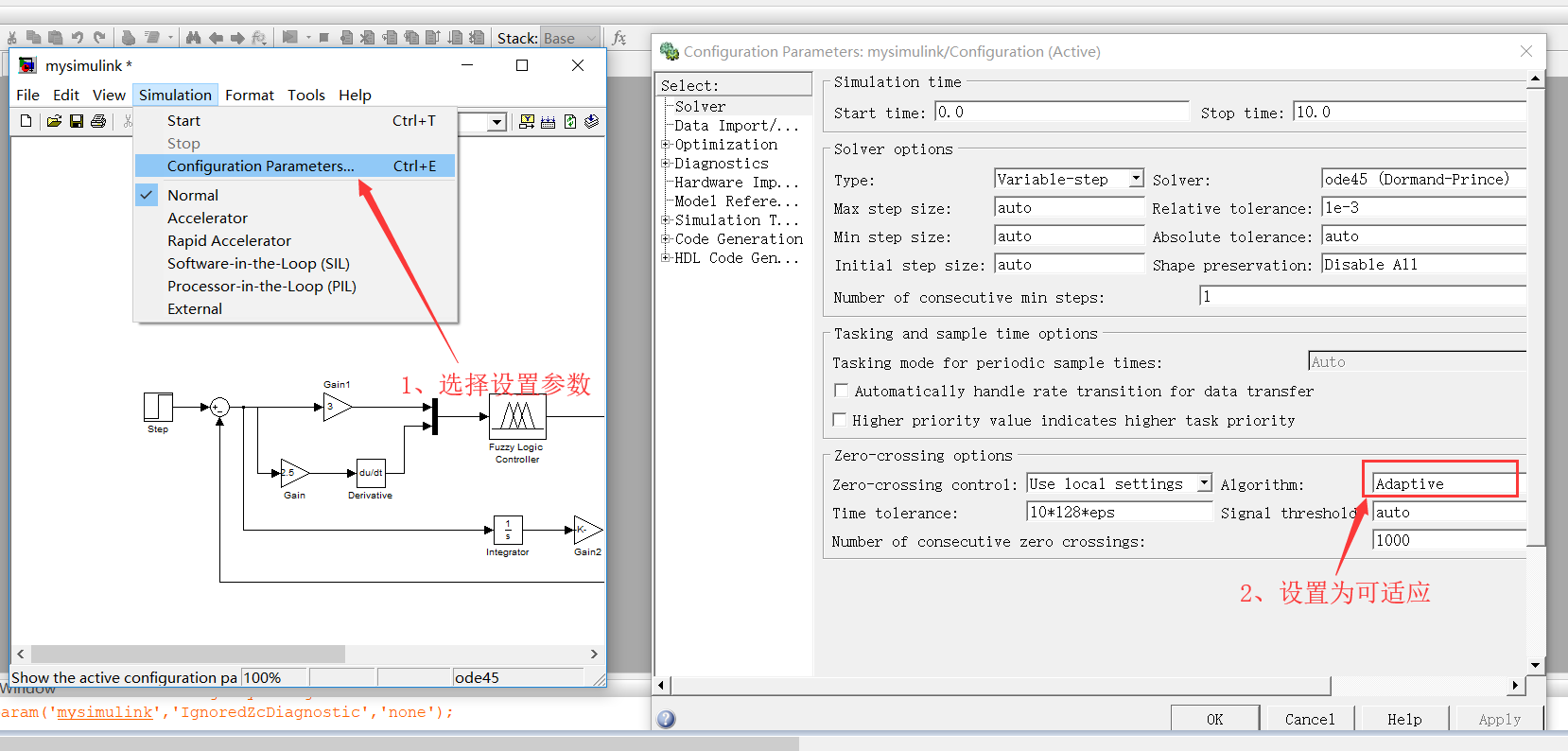开始仿真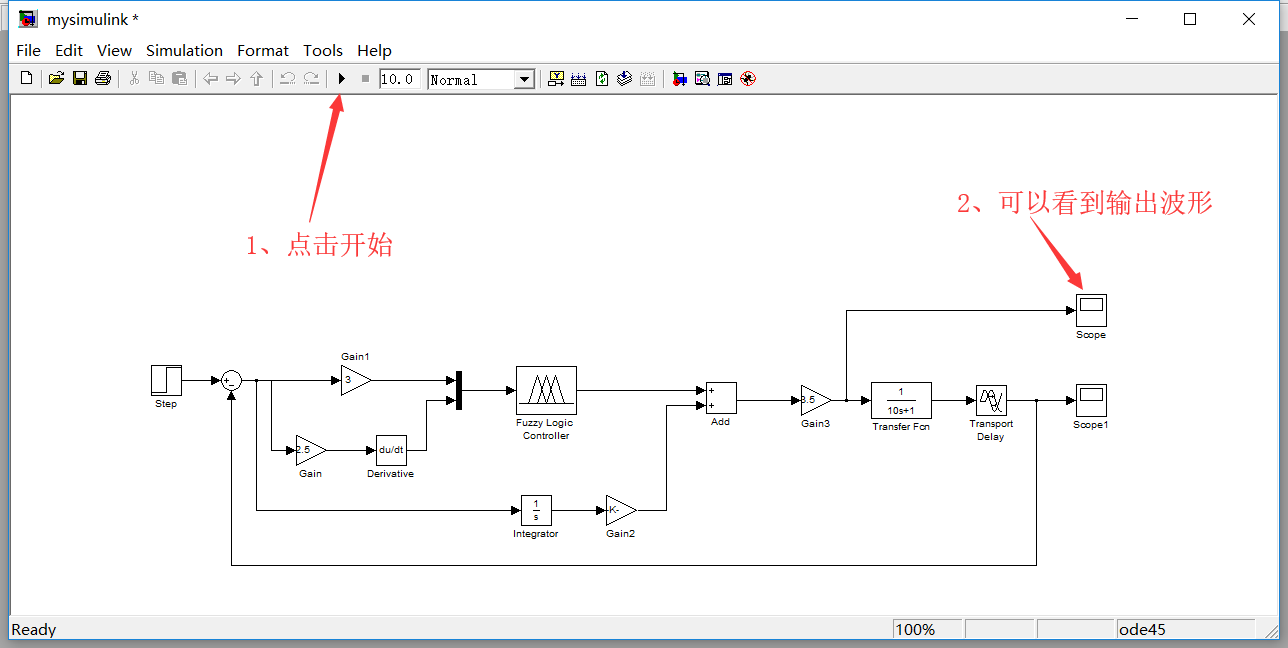展开全文• F面将根据模糊控制器设计步骤一步步利用 Matlab工具箱设计模糊控制器 Matlab模糊控制工具箱为模糊控制器的设计提供了一种非常便捷的途径 通 过它我们不需要进行复杂的模糊化模糊推理及反模糊化运算只需要设定相应参 ...
• 针对直流永磁球形电机的控制原理，提出...利用Matlab设计了球形电机控制器的上位机程序，并以DSP2407为控制器制作了球形电机控制器的硬件部分。实验结果展现了球形电机控制器的性能, 实现了球形电机洛伦兹力矢量控制。
• matlab开发-优化PID控制器设计接口。optimpid是一个基于matlab的接口，可以用来优化PID参数。
• matlab开发-nxtballbotself-balancingrobotonaball控制器设计。基于嵌入式编码器机器人NXT的NXT Ballbot模型设计。
• 基于 MATLAB 的 PID 控制器设计 一PID 控制简介 PID 控制是最早发展起来的经典控制策略, 是用于过程控制最有效的策 略之一由于其原理简单技术成在实际应用中较易于整定, 在工业控 制中得到了广泛的应用它最大的优点...
• matlab开发-内翻转摆臂的控制器设计。倒立摆控制器的设计-希伯来语
• 基于MATLAB的PID_控制器设计，较为详细的一个设计过程
• MATLAB 论文 -- 基于控制系统的 PID 调节 基于 MATLAB的 PID 控制器 摘要本论文主要研究 PID 控制器 PID 控制是迄今为止最通用的控制方法 大多数反馈回路用该方法或其较小的变形来控制 PID 控制器亦称调节器及 其...
• 建立模糊控制规则并在matlab上进行仿真，模糊控制器为两输入单输出，且模糊控制规则为5*5.
• PID 控制器设计和调整与 MATLAB 和模拟
• 以球杆系统作为主体系统中的小球作为被控对象设计一个模糊控制器控制小球在装置导轨上的运行状态分析小球在导轨上的...控制器设计并在MATLAB仿真环境下建立控制器的仿真模型对球杆系统进行仿真测试模糊控制器的控制性
• 摘 要 以球杆系统作为主体...后建立一个相对简化后的球杆系统模型 运用相关的模糊控制原理 建立球杆 系统模糊控制规则再对球杆系统进行模糊控制器设计并在 MATLAB仿真环境 下建立控制器的仿真模型对球杆系统进行仿真
• 后建立一个相对简化后的球杆系统模型运用相关的模糊控制原理建立球杆 系统模糊控制规则再对球杆系统进行模糊控制器设计并在 MATLAB 仿真环境 下建立控制器的仿真模型对球杆系统进行仿真测试模糊
• 船舶运动控制器设计仿真程序代码,MATLAB,两个M文件。
• 模糊PID控制器设计MATLAB仿真 作者:李健, 王冬青, 王丽美 作者单位:青岛大学自动化工程学院,山东,青岛,266071 刊名: 工业控制计算机 英文刊名:INDUSTRIAL CONTROL COMPUTER 年,卷(期:2011,24(5 参考文献(4条 1.......

# matlab控制器设计matlab 订阅